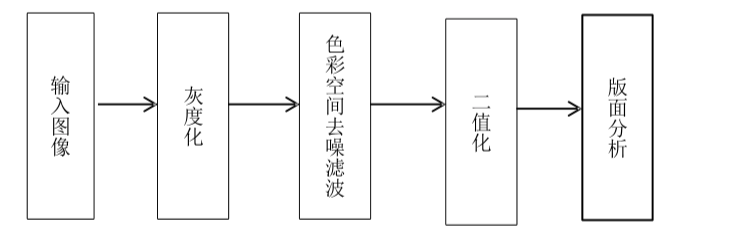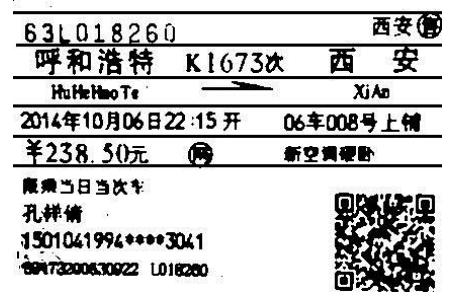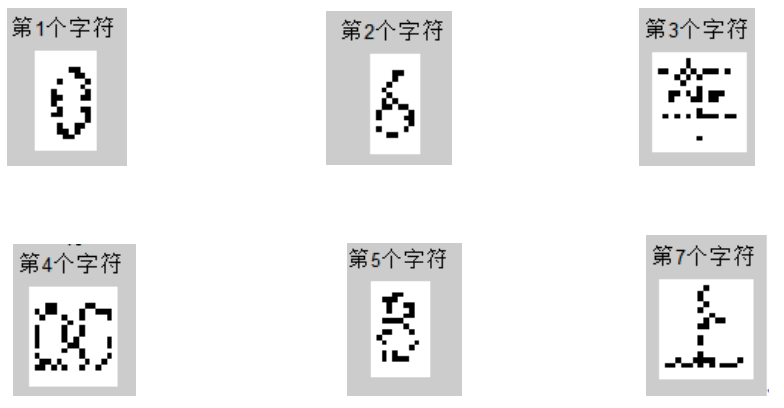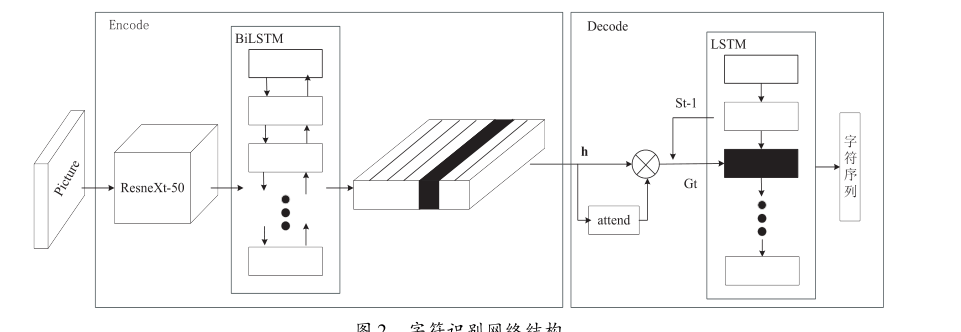• 0 前言
• 1 课题意义
• 1.1 课题难点：
• 2 实现方法
• 2.1 图像预处理
• 2.2 字符分割
• 2.3 字符识别
• 部分实现代码
• 3 实现效果

• # 0 前言

🔥 Hi，大家好，这里是丹成学长的毕设系列文章！

🔥 对毕设有任何疑问都可以问学长哦!

这两年开始，各个学校对毕设的要求越来越高，难度也越来越大… 毕业设计耗费时间，耗费精力，甚至有些题目即使是专业的老师或者硕士生也需要很长时间，所以一旦发现问题，一定要提前准备，避免到后面措手不及，草草了事。

为了大家能够顺利以及最少的精力通过毕设，学长分享优质毕业设计项目，今天要分享的新项目是

🚩 基于机器视觉的火车票识别系统

🥇学长这里给一个题目综合评分(每项满分5分)

• 难度系数：4分
• 工作量：4分
• 创新点：3分
• 🧿 选题指导, 项目分享：

https://blog.csdn.net/Mr_DC_IT/article/details/126460477

# 1 课题意义## 1.1 课题难点：

由于火车票票面文字识别属于多种字体混排，低品质的专用印刷汉子识别。火车票文字笔画粘连，断裂，识别复杂度高，难度大，采用目前较好的OCR技术都比较难以实现。

# 2 实现方法

## 2.1 图像预处理

火车票经过扫描装置火车照相机等装置将图像传递到计算机，经过灰度处理保存为一幅灰度图。如果要对火车票进行后期的识别，那么就一定要对图像做二值化，之后再对二值化的图像进行版面分析，确定我们所需要的信息所在，之后才能进行单个字符的分割，才能对字符做提取特征点的工作，之后按照我们对比确定的规则来进行判决从而达到识别效果。

由于火车票容易被污损、弯折，而且字符的颜色也是有所不同，火车票票号是红色，而其他信息显示则为黑色，票面的背景包括红色和蓝色两种彩色，这些特点都使得火车票的文字识别不同于一般的文字识别。在识前期，要对火车票图像做出特定的处理才能很好的进行后续的识别。本次课题所研究的预处理有平常所处理的二值化，平滑去噪之外还需要针对不同字符颜色来进行彩色空间上的平滑过滤。

预处理流程如下所示## 2.2 字符分割

字符分割就是在版面分析后得到的文本块切分成为文字行，之后再将行分割成单个字符，来进行后续的字符识别。这是OCR系统里至关重要的一环，直接影响识别效果。字符分割的主流方式有三种，一种是居于图像特种来寻找分割的准则，这是从结构角度进行分析切割。另一种方式是根据识别效果反馈来确认分割结果有无问题，这种方式是基于识别的切分。还有一种整体切分方式，把字符串当做整体，系统进行以词为基础的识别比并非字识别，一般这一方式要根据先验知识来进行辅助判断。

分割效果如下图所示：## 2.3 字符识别

中文/数字/英文 识别目前最高效的方法就是使用深度学习算法进行识别。

字符识别对于深度学习开发者来说是老生常谈了，这里就不在复述了；

网络可以视为编解码器结构,编码器由特征提取网络ResneXt-50和双向长短时记忆网络(BiLSTM)构成,解码器由加入注意力机制的长短时记忆网络(LSTM)构成。网络结构如下图所示。网络训练流程如下：### 部分实现代码

这里学长提供一个简单网络字符识别的训练代码：
(需要完整工程及代码的同学联系学长获取)

``````import tensorflow as tf
import numpy as np
from tensorflow.examples.tutorials.mnist import input_data
#1、开始建立一个图
sess = tf.InteractiveSession()#启动一个交互会话
x = tf.placeholder(tf.float32, shape=[None, 784])#x和y_都用一个占位符表示
y_ = tf.placeholder(tf.float32, shape=[None, 10])

W = tf.Variable(tf.zeros([784, 10]))#W和b因为需要改变，所以定义为初始化为0的变量
b = tf.Variable(tf.zeros(10))

#2、建立预测部分的操作节点
y = tf.matmul(x,W) + b  #计算wx+b
cross_entropy = tf.reduce_mean(tf.nn.softmax_cross_entropy_with_logits(labels=y_, logits=y)) #计算softmax交叉熵的均值

#3、现在已经得到了损失函数，接下来要做的就是最小化这一损失函数，这里用最常用的梯度下降做
# 为了用到前几节说过的内容，这里用学习率随训练下降的方法执行
global_step = tf.Variable(0, trainable = False)#建立一个可变数，而且这个变量在计算梯度时候不被影响,其实就是个全局变量
start_learning_rate = 0.5#这么写是为了清楚
#得到所需的学习率，学习率每100个step进行一次变化，公式为decayed_learning_rate = learning_rate * decay_rate ^(global_step / decay_steps)
learning_rate = tf.train.exponential_decay(start_learning_rate, global_step, 10, 0.9, staircase=True)

#这是因为在交互的Session下可以这样写Op.run()，还可以sess.run(tf.global_variables_initializer())
tf.global_variables_initializer().run()#初始化所有变量

#iteration = 1000, Batch_Size = 100
for _ in range(1000):
batch = mnist.train.next_batch(100)#每次选出100个数据
train_step.run(feed_dict = {x:batch, y_: batch})#给Placeholder填充数据就可以了

correct_prediction = tf.equal(tf.argmax(y,1), tf.argmax(y_,1)) #首先比较两个结果的差异
#这时的correct_prediction应该类似[True, False, True, True]，然后只要转为float的形式再求加和平均就知道准确率了
#这里的cast是用于形式转化
accuracy = tf.reduce_mean(tf.cast(correct_prediction, dtype=tf.float32))
#打印出来就可以了，注意这个时候accuracy也只是一个tensor,而且也只是一个模型的代表，还需要输入数据
print(accuracy.eval(feed_dict={x: mnist.test.images, y_: mnist.test.labels}))

sess.close()

#首先把要重复用的定义好
def weight_variable(shape):
initial = tf.truncated_normal(shape=shape, stddev=0.1)
return tf.Variable(initial)
def bias_variable(shape):
initial = tf.constant(0.1, shape=shape)#常量转变量,
return tf.Variable(initial)
def conv2d(x, f):
def max_pool_22(x):

sess = tf.InteractiveSession()#启动一个交互会话
x = tf.placeholder(tf.float32, shape=[None, 784])#x和y_都用一个占位符表示
y_ = tf.placeholder(tf.float32, shape=[None, 10])
x_image = tf.reshape(x, [-1, 28, 28, 1])
#第一层：
#1、设计卷积核1
fW1 = weight_variable([5,5,1,32])#[height, weight, in_channel, out_channel]
fb1 = bias_variable()

#2、卷积加池化
h1 = tf.nn.relu(conv2d(x_image,fW1)+ fb1)
h1_pool = max_pool_22(h1)

#第二层
fW2 = weight_variable([5,5,32,64])#[height, weight, in_channel, out_channel]
fb2 = bias_variable()

h2 = tf.nn.relu(conv2d(h1_pool,fW2)+ fb2)
h2_pool = max_pool_22(h2)

#全部变成一维全连接层，这里因为是按照官方走的，所以手动计算了经过第二层后的图片尺寸为7*7
#来定义了一个wx+b所需的w和b的尺寸，注意这里的W和b不是卷积所用的了
h2_pool_flat = tf.reshape(h2_pool, [-1, 7*7*64])#首先把数据变成行表示
W_fc1 = weight_variable([7 * 7 * 64, 1024])
b_fc1 = bias_variable()
h_fc1 = tf.nn.relu(tf.matmul(h2_pool_flat, W_fc1) + b_fc1)

#定义dropout,选择性失活,首先指定一个失活的比例
prob = tf.placeholder(tf.float32)
h_dropout = tf.nn.dropout(h_fc1, prob)

#最后一个全连接层，输出10个值，用于softmax
W_fc2 = weight_variable([1024, 10])
b_fc2 = bias_variable()
y_conv = tf.matmul(h_dropout, W_fc2) + b_fc2

cross_entropy = tf.reduce_mean(tf.nn.softmax_cross_entropy_with_logits(labels=y_, logits=y_conv))
correct_prediction = tf.equal(tf.argmax(y_conv,1), tf.argmax(y_,1))
accuracy = tf.reduce_mean(tf.cast(correct_prediction, tf.float32))

#初始化
sess.run(tf.global_variables_initializer())
for i in range(2000):
batch = mnist.train.next_batch(50)
if i%100 == 0:
train_accuracy = accuracy.eval(feed_dict = {x:batch,y_:batch, prob:1.0}) #这里是计算accuracy用的eval,不是在run一个Operation
print("step %d, training accuracy %g"%(i, train_accuracy))
train_step.run(feed_dict={x: batch, y_: batch, prob: 0.5})
print("test accuracy %g"%accuracy.eval(feed_dict={x: mnist.test.images, y_: mnist.test.labels, prob: 1.0}) )
``````

# 3 实现效果

车票图识别效果：🧿 选题指导, 项目分享：

https://blog.csdn.net/Mr_DC_IT/article/details/126460477